# Mobius strip

(diff) ← Older revision | Latest revision (diff) | Newer revision → (diff)

## Definition

The Mobius strip, or twisted cylinder, is defined as follows.

• There is a midcircle of radius$R$
• There is a half-width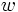$w$

Then the Mobius strip is the trace of a moving open line segment of length twice the half-width whose center traces the midcircle, and which rotates at a rate of half that at which it revolves.

## Equational descriptions

### Cartesian parametric equation

The Cartesian equation is given as follows, where$t$ varies in$[0,2\pi)$ and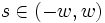$s \in (-w,w)$:

•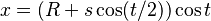$x = (R + s \cos (t/2)) \cos t$
•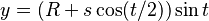$y = (R + s \cos (t/2)) \sin t$
•$z = R \sin (t/2)$

## Abstract structure

### Topological structure

Topologically, the Mobius strip can be viewed as:

$(x,y} | -1 \le y \le 1 \$

modulo the equivalence relation: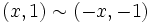$(x,1) \sim (-x,-1)$

## Related surfaces

The Mobius strip can be identified with the set of all lines in the affine plane, and hence, its one-point compatification is that set plus the line at infinity, which is the projective plane.

One approach to compactifying the Mobius strip is to identify the two bounding sides, which, locally, looks like identifying the endpoints of each line segment. This gives rise to the Klein bottle.

The Klein bottle, however, cannot be embedded in$\R^3$. It can be described topologically as follws: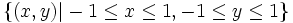$\{ (x,y) | -1 \le x \le 1, -1 \le y \le 1 \}$

modulo the following equivalence relations:$(x,1) \sim (-x,-1), (1,y) \sim (-1,y)$